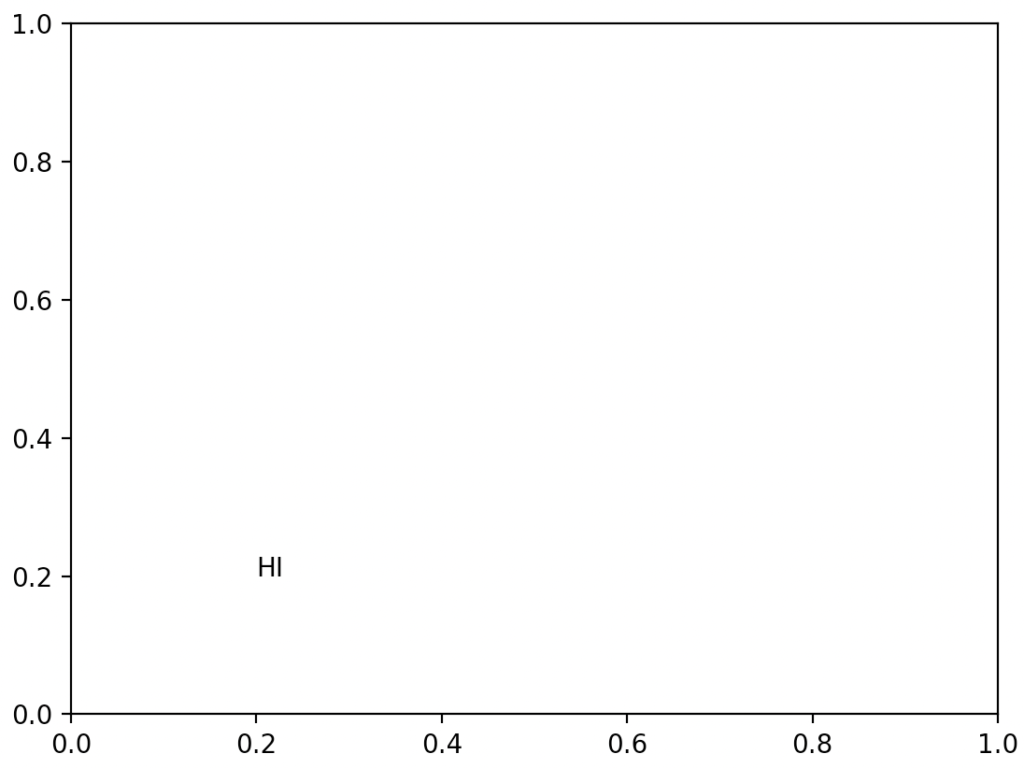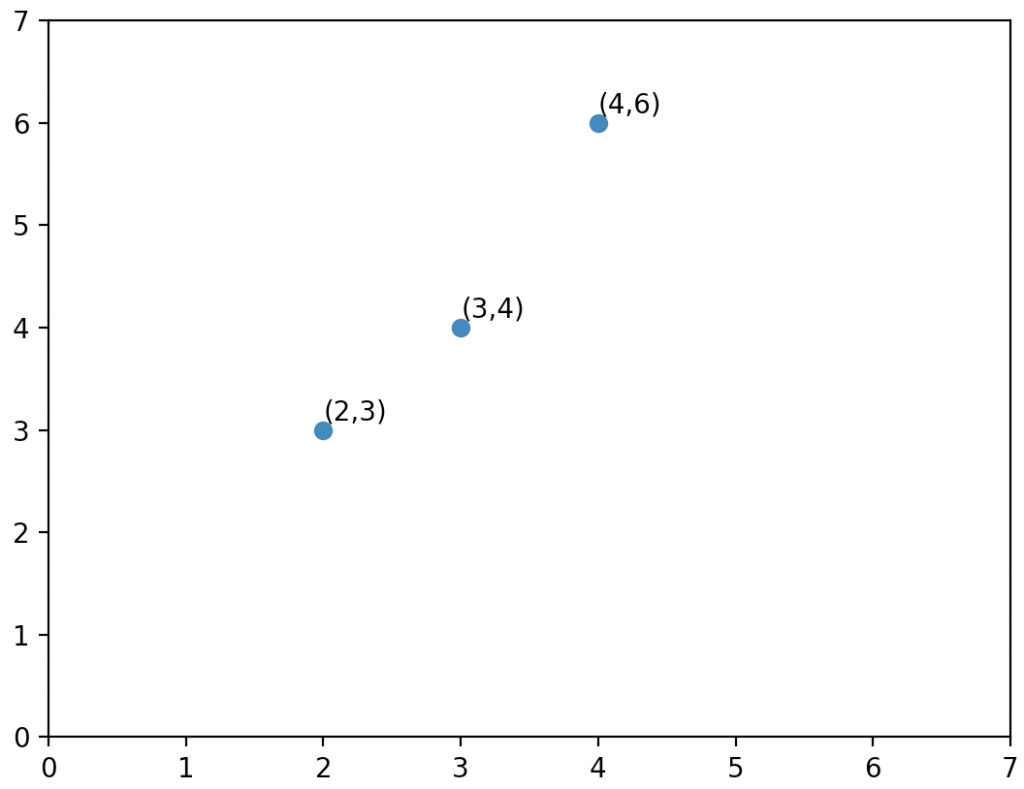# What is Matplotlib text() Function

Matplotlib.pyplot.text() is a built-in library function that adds the text to the axes.

To add text inside the plot in Python,  use the matplotlib.pyplot.text() function.

### Syntax

``matplotlib.pyplot.text(x, y, s, fontdict=None, **kwargs)``

### Arguments

The matplotlib.pyplot.text() function has three required arguments as parameters:

1. x, y: This parameter has two values, x and y. The points are passed in the x and y argument. This x and y can have values like numbers or a list of numbers. These are the required arguments.
2. s: This parameter accepts a string as a value to this argument. The text added to the plot is passed as value to this argument. This is the required argument.
3. fontdict: The text properties are given in a dictionary for this argument. If nothing is given, then the default text property is used.

### Return value

The matplotlib.pyplot.text() function returns the created text instance. The text instance is created in a graph, and that created instance is returned as output.

### Program for creating a text instance in a graph using matplotlib.pyplot.text

``````# Importing matplotlib.pyplot as plt.
import matplotlib.pyplot as plt

# Plotting the text in the point of ( 0.2, 0.2 )
plt.text(0.2, 0.2, "HI")

# Displaying the created graph
plt.show()``````

#### OutputIn this program, we imported matplotlib.pyplot for plotting the graph.

The matplotlib library consists of all the functions for plotting different types of graphs. For example, we have plotted the text HI in points 0.2 and 0.2. The text() function adds the text to the graph.

The points are passed as the first argument.

Then, the text is given. We have passed the points and the text into the text function. Then, we displayed the graph using the show function. This program plots the text Hi in the 0.2, 0.2 location.

### Program for creating a text in a line graph using matplotlib.pyplot.text

``````# Importing matplotlib.pyplot as plt.
import matplotlib.pyplot as plt

# Importing numpy as np
import numpy as np

# create a numpy array for storing the x coordinates
x = np.array([2, 3, 4])

# create a numpy array for storing the y coordinates
y = np.array([3, 4, 6])

# pass the x coordinates and y coordinates into the plot() function
plt.plot(x, y, "o")
plt.xticks([0, 1, 2, 3, 4, 5, 6, 7])
plt.yticks([0, 1, 2, 3, 4, 5, 6, 7])

# Plotting the point above each points
for i in range(len(x)):
a = "("+str(x[i])+","+str(y[i])+")"
plt.text(x[i], int(y[i])+0.1, a)

# displaying the created graph using the show method
plt.show()``````

#### OutputIn this program, we imported matplotlib.pyplot library for plotting the graph. The matplotlib library consists of all the functions for plotting different types of graphs.

We imported numpy for creating numpy arrays. We created two arrays called x and y. We have stored the x and y coordinates in these arrays.

The x array consists of 2, 3, and 4 as values. Then, the y array consists of 3, 4, and 6 values.

We plotted the points into the graph using the plot function. The plot() function takes the x and y coordinates values and then passes the format.

In this program, we passed the “o” as the format. Hence the points are plotted with the format of “o”.

The plot() function plots the points across the x and y-axis.

Then we kept the x ticks from 0 to 7 and the y ticks from 0 to 7. Then we used a for loop for iterating over the x and y points.

We created a variable for storing the points in the type of strings. First, we have created the point’s string. Then, we called the text() function. The text() function takes the x and y coordinates as a required argument.

We plotted the point 0.1 points higher than the actual point. Then, we plotted the text as points. Finally, we have displayed the graph using the show() function.

That’s it for this tutorial.

This site uses Akismet to reduce spam. Learn how your comment data is processed.